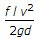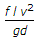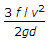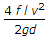# Mechanical Engineering - Hydraulics and Fluid Mechanics

### Exercise :: Hydraulics and Fluid Mechanics - Section 7

1.

The specific gravity of an oil whose specific weight is 7.85 kN/m3, is

 A. 0.8 B. 1 C. 1.2 D. 1.6

Explanation:

No answer description available for this question. Let us discuss.

2.

The body will float if the force of buoyancy is __________ the weight of the liquid displaced.

 A. equal to B. less than C. more than

Explanation:

No answer description available for this question. Let us discuss.

3.

According to Darcy's formula, the loss of head due to friction in the pipe is (where f = Darcy's coefficient, l = Length of pipe, v = Velocity of liquid in pipe, and d = Diameter of pipe)

 A.B.C.D.Explanation:

No answer description available for this question. Let us discuss.

4.

The ratio of specific weight of a liquid to the specific weight of pure water at a standard temperature is called

 A. density of liquid B. specific gravity of liquid C. compressibility of liquid D. surface tension of liquid

Explanation:

No answer description available for this question. Let us discuss.

5.

The velocity of liquid flowing through an orifice varies with the available head of the liquid.

 A. Agree B. Disagree